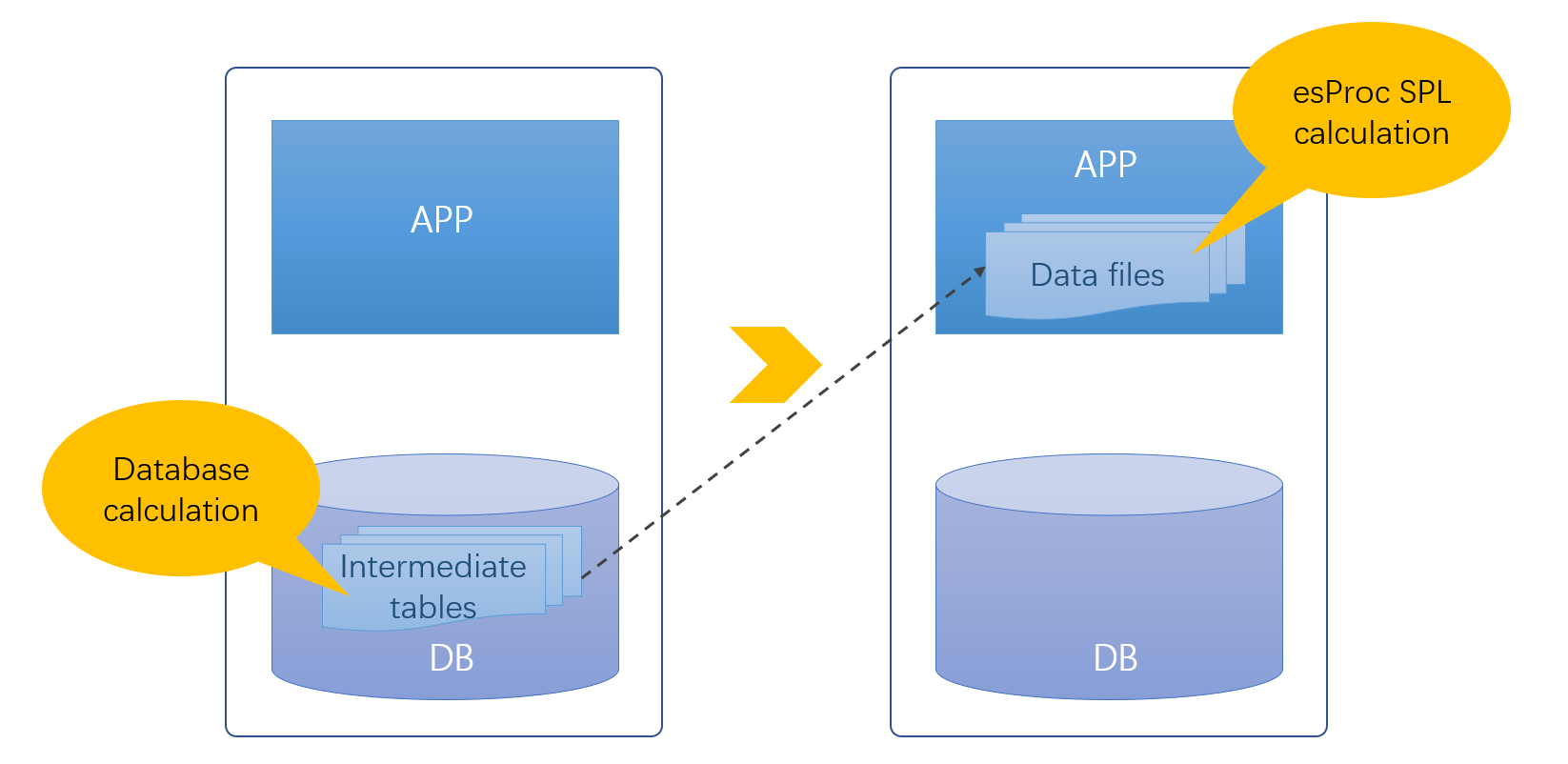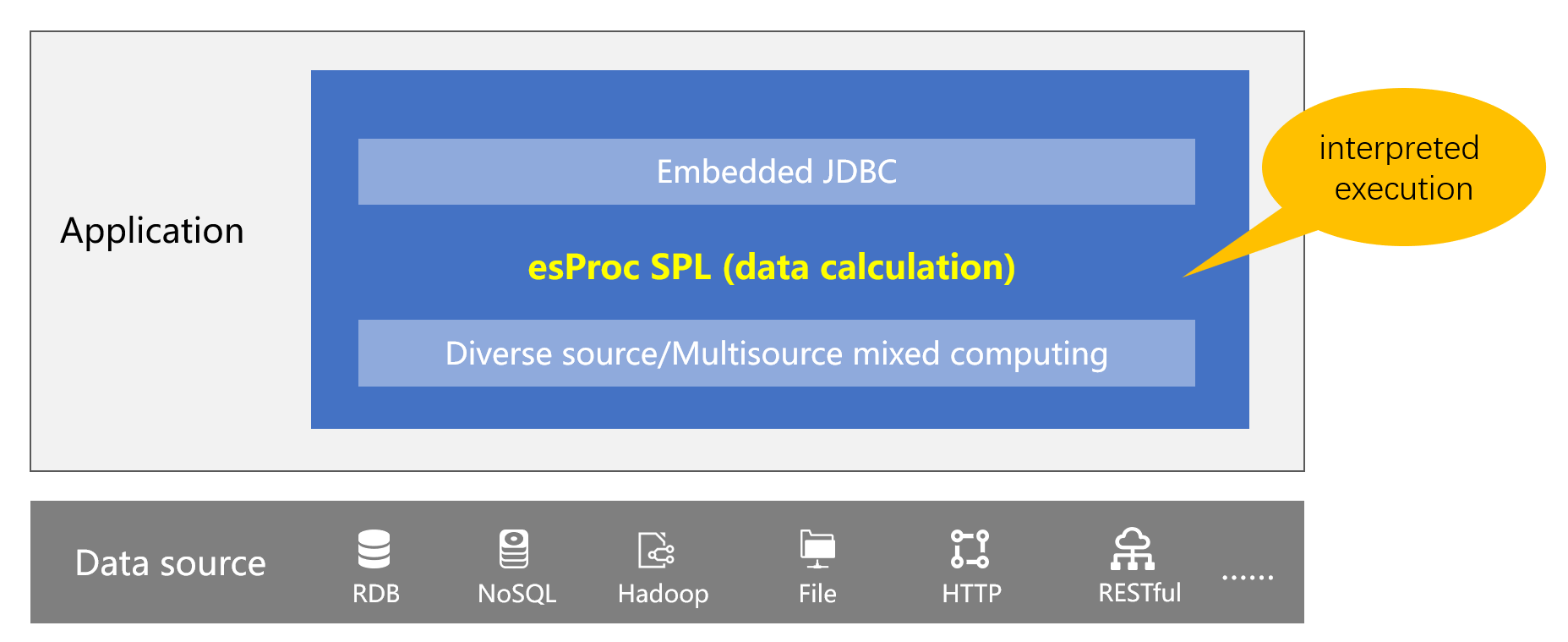# 数据库中为什么会有这么多中间表？## 文件计算

SPL 可以直接基于文件（Excel、CSV、JSON、SPL 二进制）计算，原有的数据库中间表可以直接存储在库外文件中，充分减少数据库中间表数量，缓解数据库压力。

## SPL 敏捷语法

 A 1 =file(“/opt/ods/orders.csv”).import@tc() 2 =A1.groups(customer;sum(amount):amount).sort(amount:-1) 3 =A2.sum(amount)/2 4 =0 5 =A2.pselect((A4=A4+amount,A4>=A3)) 6 =A2.(customer).to(,A5) 7 =A1.select(A6.pos(A1.customer))

SPL 解释执行，支持热部署。

## 高性能